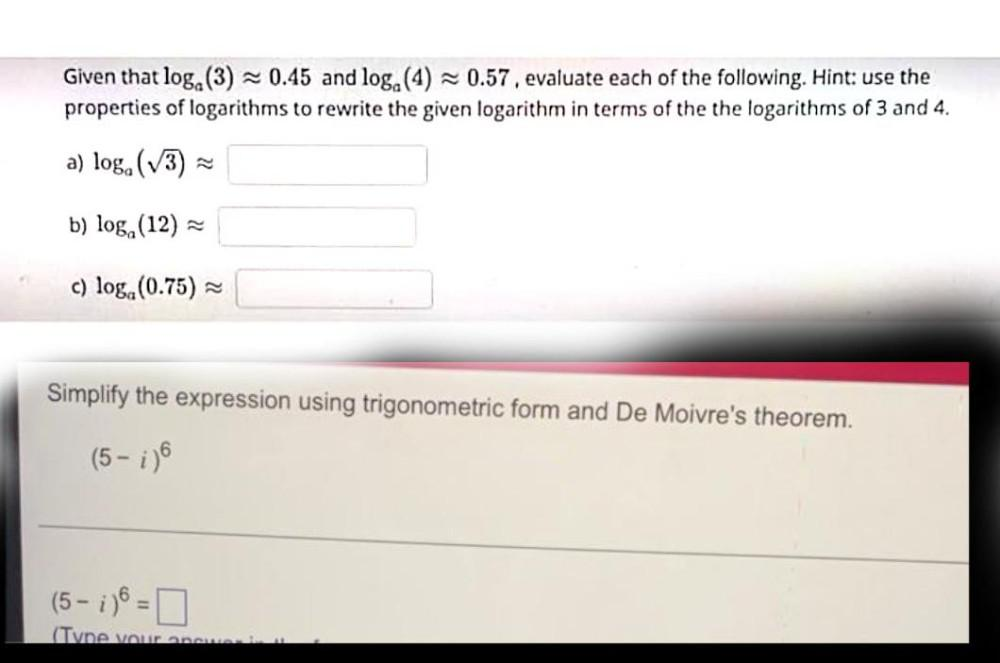Question:

# Given that log.(3) = 0.45 and log.(4) -0.57. evaluate each of the following. Hint: use the properties of logarithms to rewrite tGiven that log.(3) = 0.45 and log.(4) -0.57. evaluate each of the following. Hint: use the properties of logarithms to rewrite the given logarithm in terms of the the logarithms of 3 and 4. a) loga (3) b) loga (12) c) log.(0.75) = Simplify the expression using trigonometric form and De Moivre's theorem. (5 - ;) (5-1) = 0 (Tvne vou